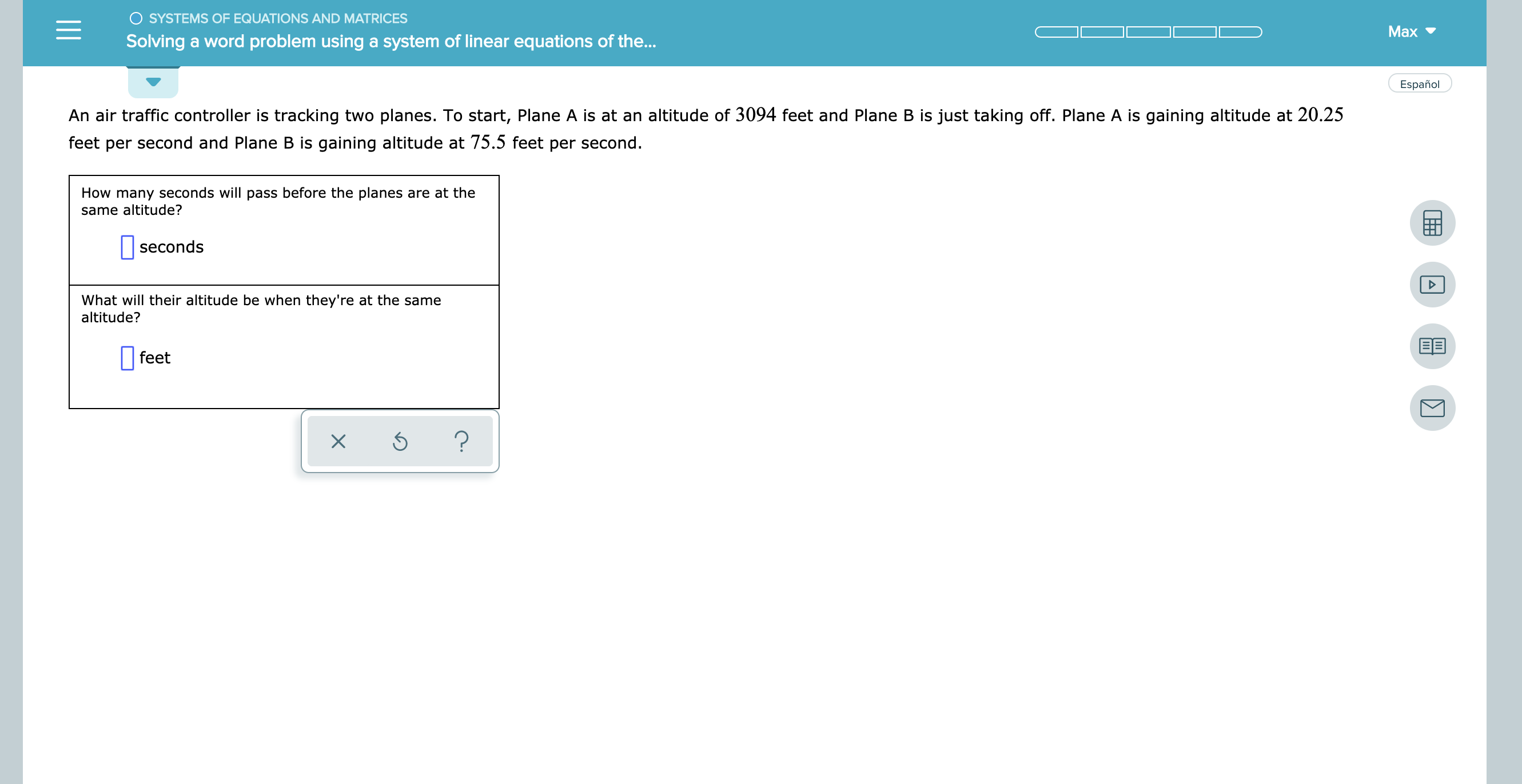# SYSTEMS OF EQUATIONS AND MATRICES Max Solving a word problem using a system of linear equations of the... Español An air traffic controller is tracking two planes. To start, Plane A is at an altitude of 3094 feet and Plane B is just taking off. Plane A is gaining altitude at 20.25 feet per second and Plane B is gaining altitude at 75.5 feet per second How many seconds will pass before the planes are at the same altitude? seconds What will their altitude be when they're at the same altitude? feet ? X

Question

see attachmenthelp_outlineImage TranscriptioncloseSYSTEMS OF EQUATIONS AND MATRICES Max Solving a word problem using a system of linear equations of the... Español An air traffic controller is tracking two planes. To start, Plane A is at an altitude of 3094 feet and Plane B is just taking off. Plane A is gaining altitude at 20.25 feet per second and Plane B is gaining altitude at 75.5 feet per second How many seconds will pass before the planes are at the same altitude? seconds What will their altitude be when they're at the same altitude? feet ? X fullscreen

### Want to see this answer and more?

Experts are waiting 24/7 to provide step-by-step solutions in as fast as 30 minutes!*

*Response times vary by subject and question complexity. Median response time is 34 minutes and may be longer for new subjects.
Tagged in
MathCalculus

### Other